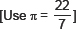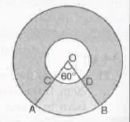# Areas related to Cirles-Sample Questions

Class X Math
Sample paper for Areas Related to Circles
1.   The area of a n equilateral triangle is 49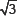cm2. Taking each angular point as centre, circles are drawn with radius equal to half length of the side of the triangle. Find the area of triangle not included in the circles.
2.   A square OABC is inscribed in a quadrant OABQ of a circle as shown in the figure. If OA = 14 cm, find the area of the shaded region.
3.   In the figure, ABDC is a quadrant of a circle of radius 14 cm and a semi-circle is drawn with diameter BC. Find the area of the shaded region.
4.   Four equal circle are described about the four corners of a square so that each touches two of the others, as shown in teh figure. Find the area of the shaded region, each side of the square measuring 14 cm.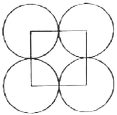5.   In the figure, MN and PQ are teh arcs of two concentric circles of radii 7 cm and 3.5 cm respectively and ∠MON = 30°. Find the area of the shaded region.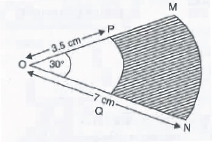6.   A play ground is in the form of a rectangle havign semi-circles on the shorter sides as shown in the figure. Find its area when the length of the rectangular portion is 38 m and the breadth is 10 m. [use π = 3.14]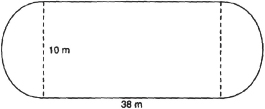7.   A bicycle wheel makes revolutions per minute to maintain a speed of 8.91 km per hour.
Find the diameter of the wheel.
8.   Find the area of the shaded region in the given figure, if PQ = 24 cm, PR = 7 cm and O is the centre of the circle.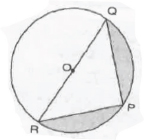9.   In the figure, from a rectangular region ABCD with AB = 20 cm, a right raignale AED with AE = 9 cm and DE = 12 cm, is cut off. On the other end, taking BC as diameter, a semi-circle is added on outside the region. Find the area of the shaded region.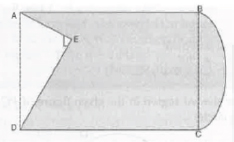10.   In the figure, two concentric circles with centre O, have radii 21 cm and 42 cm. If ∠AOB = 60°, find the area of the shaded region.